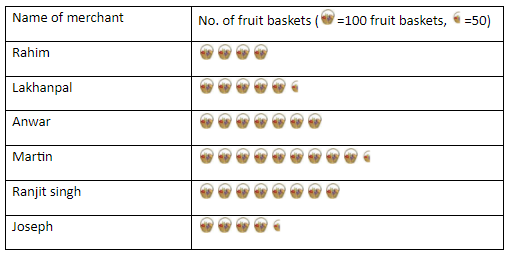QuestionAnswers

# In a village, six fruit merchants solve the following number of fruit baskets in a particular session.Observe the pictograph and answer the following questions:(a) Which merchant sold the maximum number of baskets?(b) How many fruit baskets were sold by Anwar?(c)The merchants who have sold 600 or more number of baskets are planning to buy a godown for      the next season. Can you name them?Verified
129k+ views
Hint: Converting given pictorial data (here data = each basket) with the corresponding number 100 and half basket with 50.

Convert each basket against each merchant with the corresponding number to obtain the fruit baskets sold by each, where the complete basket represents 100 fruit baskets and half fruit basket represents 50 fruit baskets.
Number of fruit baskets of each merchant:
Rahim = $4 \times 100 = 400$ (4 full picture each of 100 baskets),
Lakhanpal = $(5 \times 100) + (1 \times 50) = 550$ (5 full picture each with 100 baskets and one with 50),
Anwar = $7 \times 100 = 700$ , (7 full picture each of 100 baskets),
Martin = $(9 \times 100) + (1 \times 50) = 950$, (9 full picture each with 100 baskets and one with 50),
Ranjit Singh = $8 \times 100 = 800$ , (8 full picture each of 100 baskets),
Joseph = $(4 \times 100) + (1 \times 50) = 450$, (4 full picture each with 100 baskets and one with 50),
(a) Merchant Martin sold 800 which is the maximum number of fruit baskets among all the other merchants.
Total number of baskets $= 400 + 550 + 700 + 950 + 800 + 450 = 3850$